# How to Add 10 and 100 to a Number

How to Add 10 and 100 to a Number#### How to Add 10 to a Number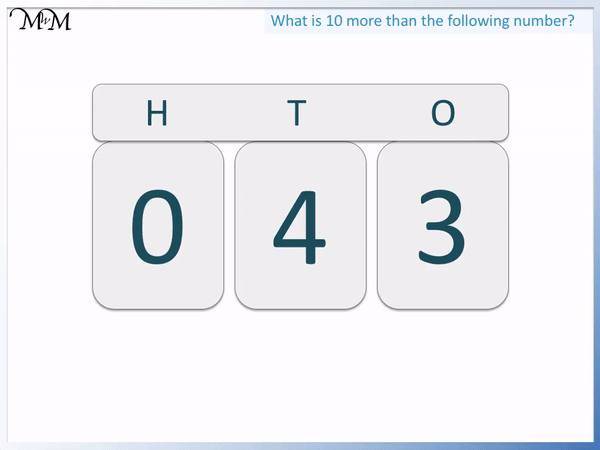• To add 10 to a number, increase the digit in the tens column by 1.
• The tens column is the second column from the right.
• Leave all other digits the same.
• The digit of ‘4’ in 43 increases by 1 to become ‘5’.
• 43 + 10 = 53.

#### How to Add 100 to a Number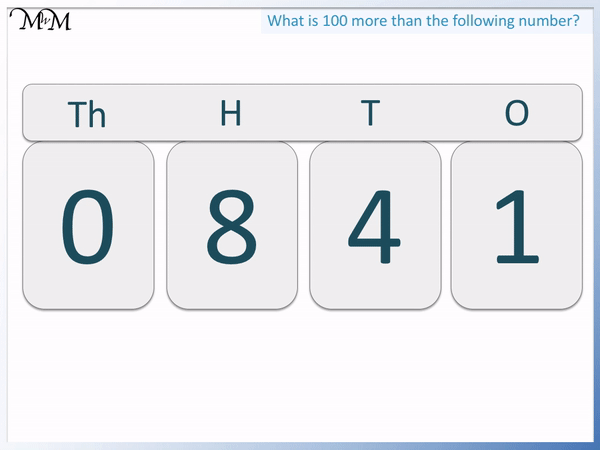• To add 100 to a number, increase the digit in the hundreds column by 1.
• The hundreds column is the third column from the right.
• Leave all other digits the same.
• The digit of ‘8’ in 841 increases by 1 to become ‘9’.
• 841 + 100 = 941.

To add 10 to a number, increase the tens column digit by 1.

To add 100 to a number, increase the hundreds column digit by 1• We are adding 100 to the number 3951.
• We increase the digit in the hundreds column by 1.
• 9 is the largest digit on our flipchart.
• So we instead think of 3951 as having ’39’ hundreds.
• We increase ’39’ hundreds by 1 to get ’40’ hundreds.
• 3951 + 100 – 4051.#### Adding 100 using a Flipchart#### Interactive Place Value Flipchart

Here is an online place value flipchart to help teach adding 10 and 100.

## How to Add 10 to a Number

To add 10 to a number, increase the tens digit by 1 and keep all other digits the same. If the tens digit is a 9, then change the 9 to a 0 and increase the hundreds digit by 1.

For example, here is 43. The tens digit is the digit that is second from the right. The tens digit of 43 is ‘4’.

To add 10 to 43, we increase the tens digit by 1.

4 + 1 = 5 and so, the tens digit increases from 4 to 5.43 + 10 = 53.

In this example, we are adding 10 to 18.

We increase the tens digit by 1.

The tens digit of 18 is ‘1’.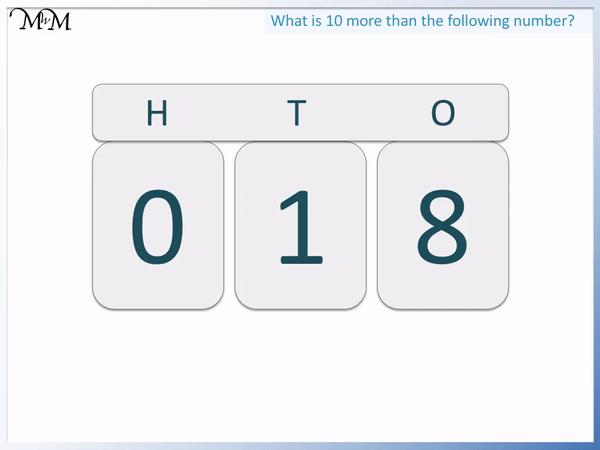1 + 1 = 2 and so, 18 + 10 = 28.

We can see in these examples that when adding 10, we only changed the tens digit. All other digits in the number remained the same.

This is true when adding 10 to larger numbers too.

Here is 457 + 10.

The tens digit is ‘5’, so we increase 5 to 6.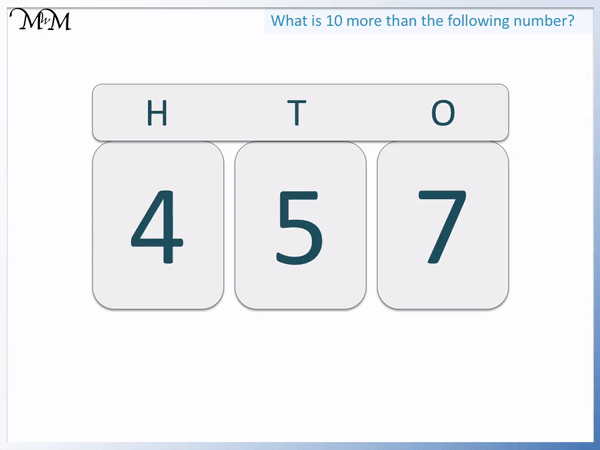457 + 10 = 467

We can see that both the digits of ‘4’ and ‘7’ remained the same.

We need to be more careful when adding ten to a number is when the tens digit is a 9.

Here is an example of 92 + 10.We currently have a ‘9’ in the tens column. We can increase 9 by 1 to make 10.

We now have 10 tens. To write this, the 9 becomes a 0 and we put a 1 in the hundreds column.

This is because ten tens are one hundred.

In this next example, we have 195 + 10.

Again, we have a ‘9’ in the tens column. We can think of 195 as having ’19’ tens and 5 ones.

We increase 19 by 1 to make 20 tens.The 19 becomes 20 tens and the 5 ones remain the same.

195 + 10 = 205

We can see that if the digit in the tens column is a 9, then to add 10, we change the 9 to a 0 and increase the hundreds column by 1 instead.

## How to Add 100 to a Number

To add 100 to a number, increase the hundreds digit by 1. If the hundreds digit is a 9, then change the 9 to a 0 and increase the thousands digit by 1 instead.

Here is a place value flipchart showing 28. We will count up in hundreds by adding 100 each time. To add 100, we increase the digit in the hundreds column by 1.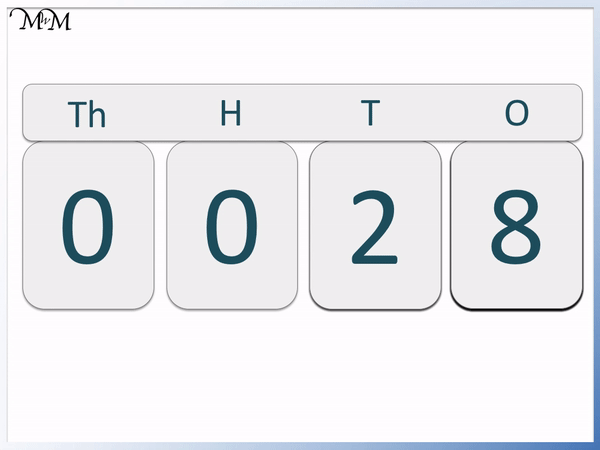Starting with 28, we have 0 hundreds.

We increase this to 128 by adding 100.

Each time 100 is added, we increase the digit in the hundreds column until we reach 928.

To add 100 to 928, we change the 9 to a 0 and increase the thousands digit by 1.

The thousands digit increases from 0 to 1.

928 + 100 = 1028

It is easiest to think of 928 as having 9 hundreds and after adding 100, 1028 has 10 hundreds.

Here is an example of finding 100 more than a number.

To find 100 more than a number, simply increase the digit in the hundreds column by 1.

We want to find one hundred more than 106. 106 has a ‘1’ in the hundreds column.We increase the ‘1’ in 106 to a ‘2’.

106 + 100 = 206

206 is one hundred more than 106.

To find one hundred more than a number, we must add 1 to the hundreds digit but keep all other digits the same.

In this example we are finding 100 more than 1548.

‘5’ is the hundreds digit.We increase the ‘5’ to a ‘6’ but keep all other digits the same.

1548 + 100 = 1648

We can see that the digits of ‘1’, ‘4’ and ‘8’ in the other place value columns remained the same.

We need to be more careful when finding 100 more than a number that has a digit of 9 in the hundreds column.

In this example we are adding 100 to 970, which has a 9 in the hundreds column.

We increase the 9 to a 10.

However, to write 10, we change the 9 into a 0 and put a 1 in the next column along.970 + 100 = 1070

Here is another example of finding 100 more than a number that has a 9 in the hundreds column.

We have 3951.

Because the hundreds column digit is a 9, we change the 9 to a 0 and increase the thousands column digit by 1.

3951 + 100 = 4051We can think of the number 3951 as having ’39’ hundreds.

When we add 1 to 39, we get 40.

The answer of 4051 has 40 hundreds and so, we have still increased by 1 hundred.

## How to Teach 10 More than a Number

To teach 10 more than a number, it is best to use a place value flipchart. Practise with a variety of different numbers, showing how the tens digit increases by 1 each time 10 is added.

Here is a link to our free interactive place value flipchart for practising finding 10 more than a number.

It is helpful to teach adding 10 by starting with a small number and cycling through the digits. You should say each number as you add ten and change the digit, getting your child to say the number as well.

It is sensible to teach counting up in ones before teaching counting up in tens. It is helpful to familiarise the child with the flipchart by first counting up in ones.

Here we start with 6 on the flipchart. We keep adding 10 to find ten more than the previous number.

The pattern should become clear.We add 10 by increasing the tens digit of the flipchart.We can also teach finding 10 more than a number using a number grid.

To find 10 more than a number, simply move one place down on the grid.

For example, here we start with 32 and add 10 to find the number that is 10 more.

42 is directly below 32 and so, it is 10 more.It is helpful to use a variety of methods to teach each concept and both the flipchart and number grid can be used to teach finding 10 more than a number.Now try our lesson Adding 3 Single Digit Numbers where we learn how to add 3 numbers together.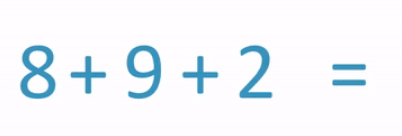error: Content is protected !!